Pressure and Total Force

# 6 Pressure and Force

Click play on the following audio player to listen along as you read this section.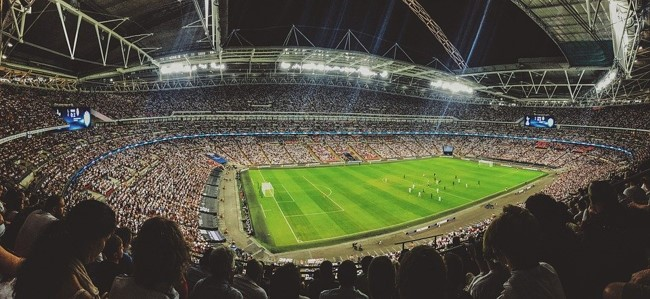Imagine you are playing in the World Cup of Soccer and you line up to take a penalty kick. If you score your team wins but if you miss you won’t be the most popular person in your home country. Would you feel pressure? How would you describe that pressure?

Thankfully we’re not going to talk about that kind of pressure in this section. We are going to talk about the pressure exerted from one object or source to another. Take a look at the following picture to see an example of pressure.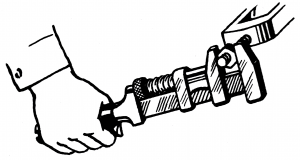In the picture above a person is applying pressure to the wrench in order to turn the nut on the bolt. Force also comes into play here and is a very similar concept. This chapter will talk about both pressure and force and the relationship between the two.

# The Difference Between Pressure and Force

If you have ever used the words pressure and force interchangeably you are not alone. For many of us the words mean the same thing but in fact there is a difference between them. Here’s the quick and easy version of how the two words differ.

Force is the total impact of one object on another while pressure is the ratio of that force to the area over which it is applied.

What we have is two concepts that are indeed very similar but at the same time have some differences. Take a look at the picture below.The hardest slapshot every recorded was by defenseman Zdeno Chara when he was with the Boston Bruins of the National Hockey League. He shot the puck an incredible 108.8 miles per hour. Not bad!

Now in order to move the puck from stationary to 108.8 mph he had to apply force to the puck.

Force is defined as a push or a pull that makes an object change its state of motion. In this case Zdeno created a very large push to get the puck moving that fast.

So right about now you might be asking, “Well, if that is force, then what is pressure?” Could we say that Zdeno put a lot of pressure into the shot? In fact he did. As stated earlier, pressure is very similar to force.

Pressure is the force over a certain area. Basically pressure is force per unit area.

Pressure = force per unit area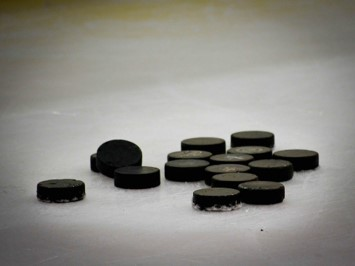How much of the puck do you think comes into contact with the stick? Whatever that area is it would be the area that the force is transferred to.

What we say is that there is a certain amount of pressure applied to the puck. The most common example of pressure we would find in the trades would be pounds per square inch. When the player strikes the puck he/she transfers the energy from the stick to the puck.

Pressure: A force over a defined area

Example: pounds per square inch (psi)

If we can determine that the puck has had a certain amount of pounds per square inch exerted on it, then to calculate force we just need to find the area that the pressure is transferred onto to the puck. It is preferable that this area be in square inches as this will work well with a pressure in pounds per square inch.

Force: Takes the pressure and applies it to the whole area of the object that is affected.

Take a look at the picture below for one last clarification.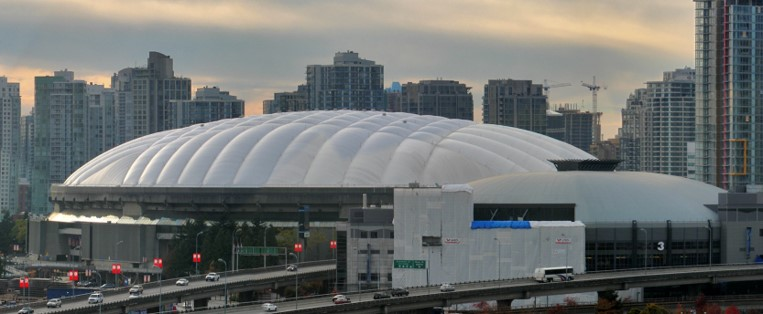The picture is of BC Place Stadium in downtown Vancouver. It shows the roof that was originally on before it was changed to the new “open and close” concept roof.

The interesting thing about this roof is that it was kept inflated by pressure. As it turns out the pressure on the inside of the stadium was just slightly higher than the pressure on the outside of the stadium, thus keeping the roof up.

This difference of pressure can be as little as 0.037 psi. Therefore the pressure exerted on the inside of the roof is 0.037 pounds on every square inch of the roof.

The total force is simply the amount of pressure exerted on one square inch and then multiplied by how many square inches there are on the inside of the roof.

This small pressure difference from the outside of the roof to the inside of the roof is enough to keep the roof inflated.

Now that we have an idea what pressure is how do we calculate it? Well the easiest way to calculate is to use a pressure gauge.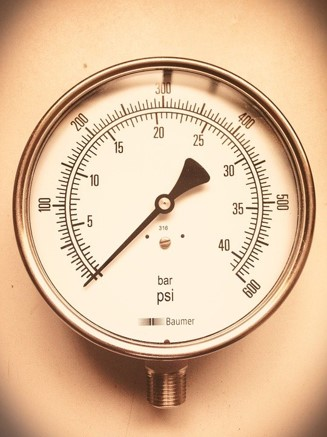The above picture is an example of a pressure gauge rated in pounds per square inch. If you were to attach this gauge to a piping system it would indicate the pressure of the liquid or gas in that system above atmospheric pressure.

Pounds per square in not the only way we measure pressure. Below are three of the more common units used when dealing with pressure.

Pounds per square inch (psi)

Newton per square meter (AKA pascal) (N/m2)

Kilograms per square centimeter (Kg/cm2)

Remember that pressure is a force on a unit of area.

As stated before we don’t physically calculate pressure ourselves but we do it with the help of a pressure gauge. In the piping field we are generally dealing with the pressure of liquids or gases inside containers or pipes.

For example a compressed gas cylinder filled with medical gas might be filled to 2000 psi or a water system in a house might be tested to 200 psi. We would know this by looking at a pressure gauge attached to the cylinder or piping system.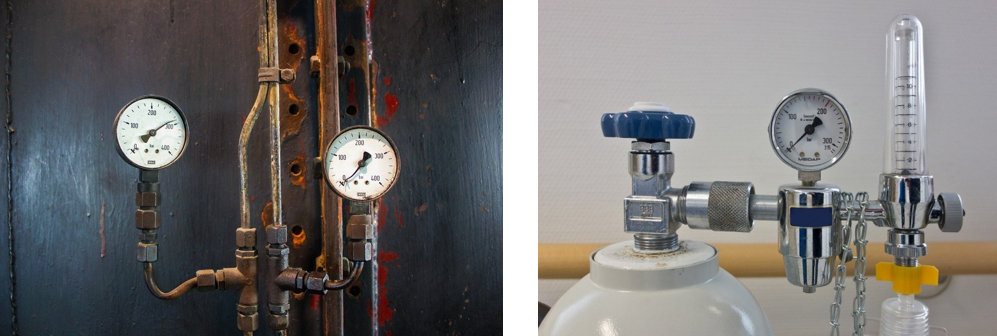So in summary we have two concepts. One is force which is the amount of push of pull on an object. We then have pressure. That’s when we take the force and apply it to a particular area. In the next section we will take a look at how water plays a role in determining pressure and ultimately force.